Hypergeometric Distribution
 Poisson Distribution Geometric Distribution Exam Engineered Software Home Page The hypergeometric distribution is similar to the binomial distribution. Both are used to model the number of successes given a fixed number of trials, and two possible outcomes on each trial. The difference is that the binomial distribution requires the probability of success to be the same for all trials, while the hypergeometric distribution does not. Consider drawing from a deck of cards. If 5 cards are drawn, the probability of getting exactly 2 harts can be computed using the binomial distribution if after each draw the card is replaced in the deck and the cards are shuffled. By replacing the card and shuffling, the probability of getting a hart on each of the 5 draws remains fixed at 13/52. If the card is not replaced after each draw, the probability of getting a hart on the first draw is 13/52, but the probability of getting a hart on the second draw is dependent on the outcome of the first draw. If the first draw resulted in a hart, the probability of getting a hart on the second draw is 12/51. If the first draw did not result in a hart, the probability of getting a hart on the second draw is 13/51. The hypergeometric distribution is used to model this situation. This is also why the hypergeometric distribution is referred to as the distribution that models sampling without replacement. The hypergeometric probability density function is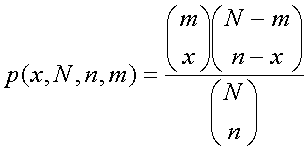where p(x,N,n,m) is the probability of exactly x successes in a sample of n drawn from a population of N containing m successes.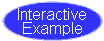The hypergeometric cumulative distribution function is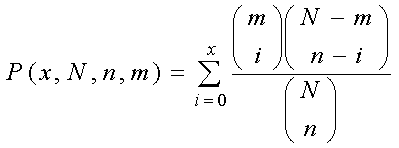The mean and the variance of the hypergeometric distribution are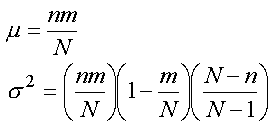Example Fifty items are submitted for acceptance. If it is known that there are 4 defective items in the lot, what is the probability of finding exactly 1 defective item in a sample of 5? What is the probability of finding less than 2 defective items in a sample of 5? Solution For this example, x = 1 N = 50 n = 5 m = 4 The probability of finding exactly 1 defective item in a sample of 5 is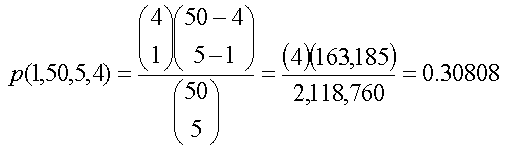This answer can be found using the Excel function =HYPGEOMDIST(1,5,4,50) The probability of finding less than 2 defective items in a sample of 5 is equal to the probability of exactly zero plus the probability of exactly 1. The probability of exactly zero is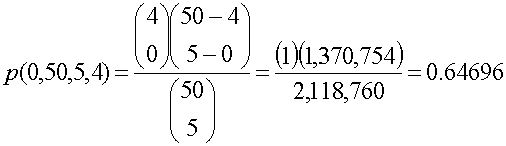The probability of finding less than 2 defective items in a sample of 5 is 0.30808 + 0.64696 = 0.95504. Unfortunately Excel does not have a cumulative form of the hypergeometric distribution.  Click Here to download this solution in Microsoft Excel. The binomial distribution can be used to approximate the hypergeometric distribution when the population is large with respect to the sample size. When N is much larger than n, the change in the probability of success on a single trial is too small to significantly effect the results of the calculations. Again, it is silly to use approximations when exact solutions can be found with electronic spreadsheets. These approximations were useful for the engineer toiling with a slide rule, but are of little use now.